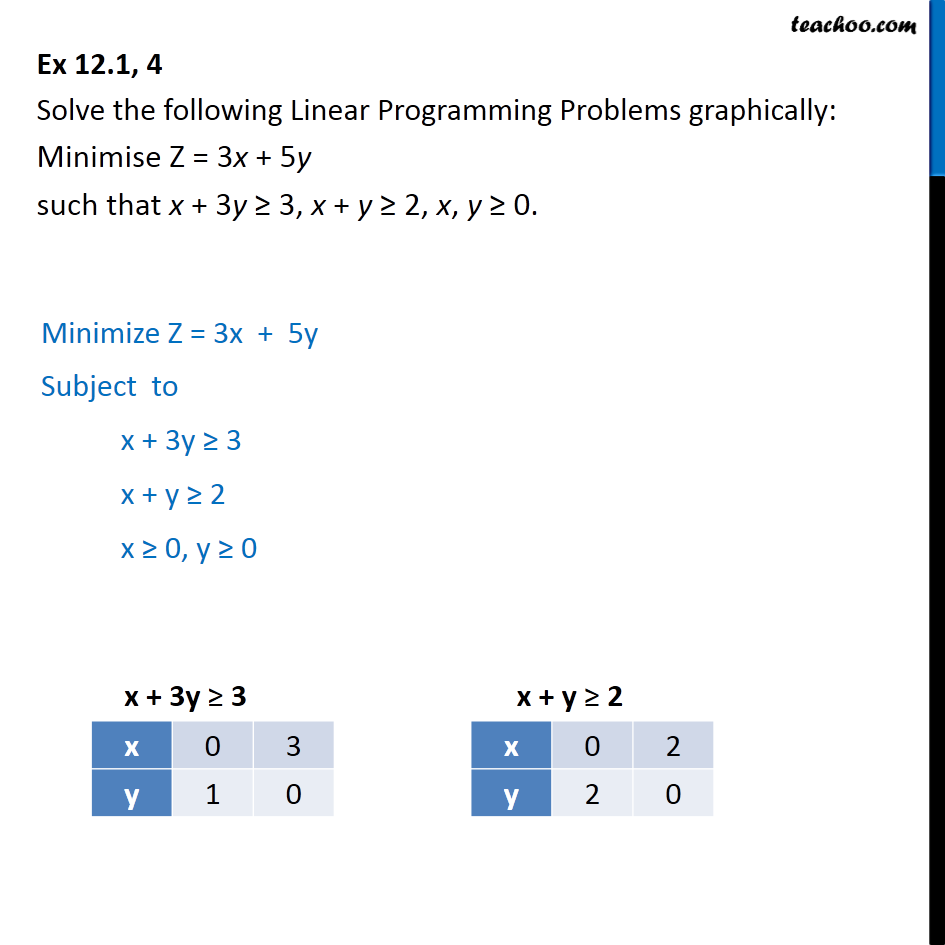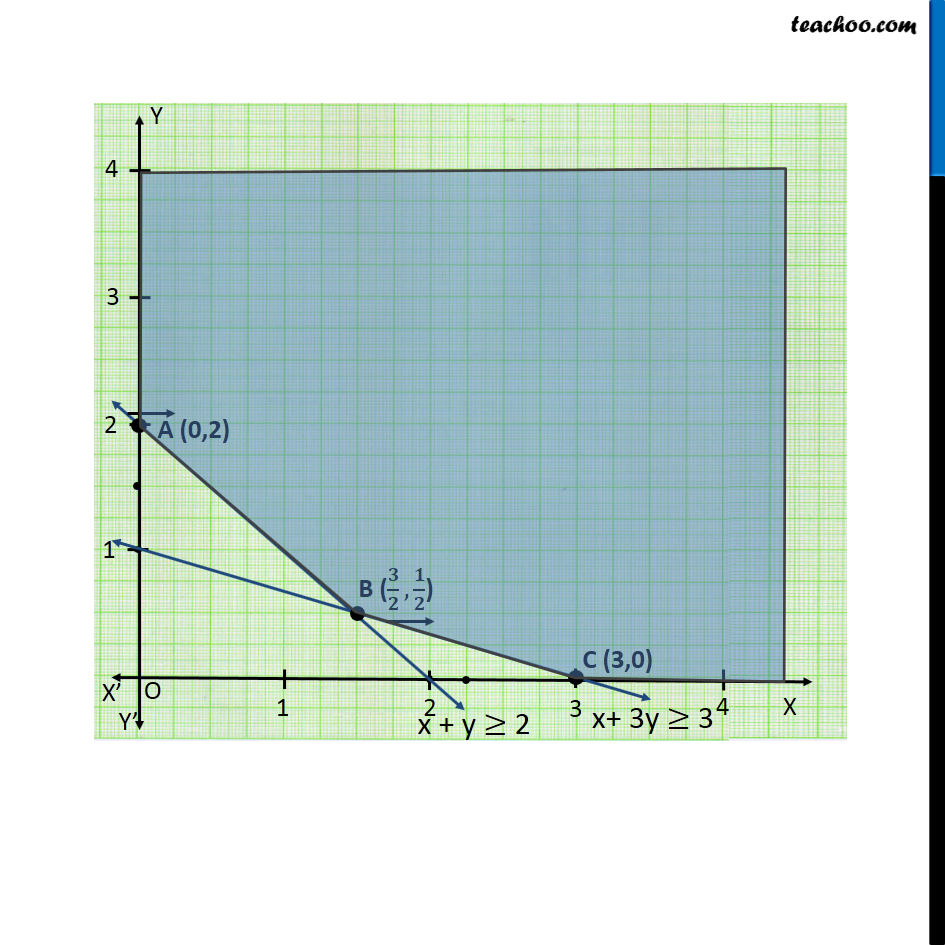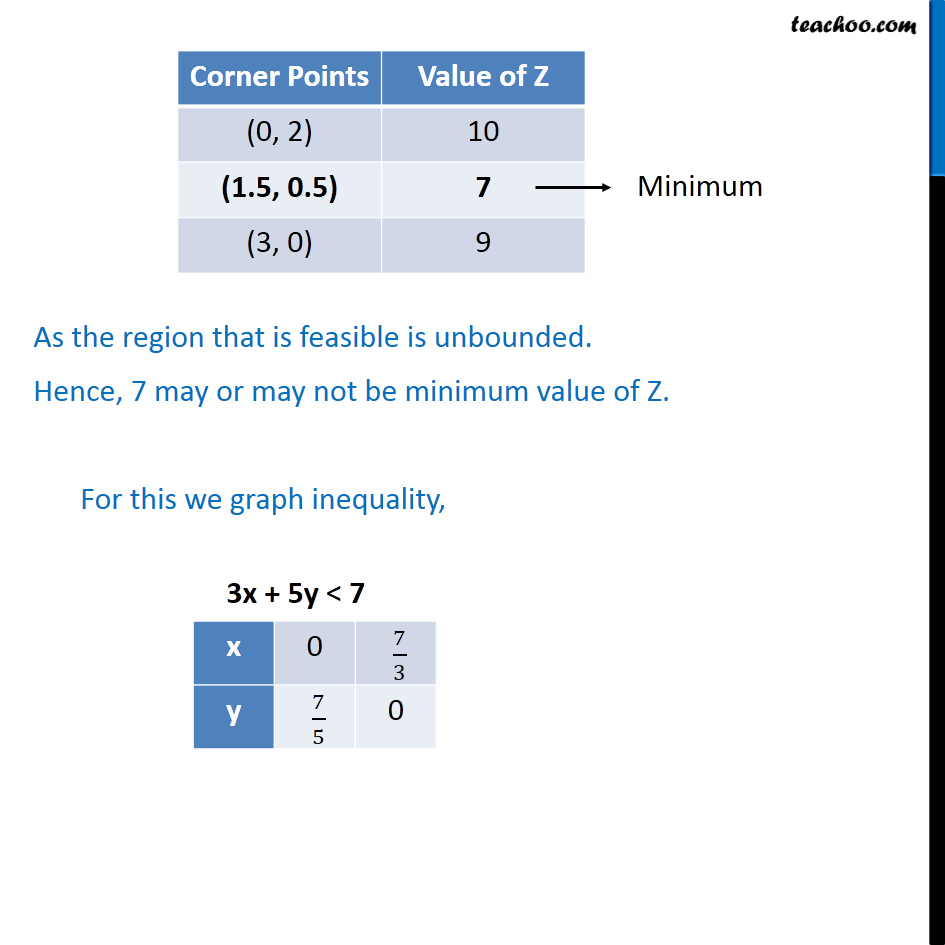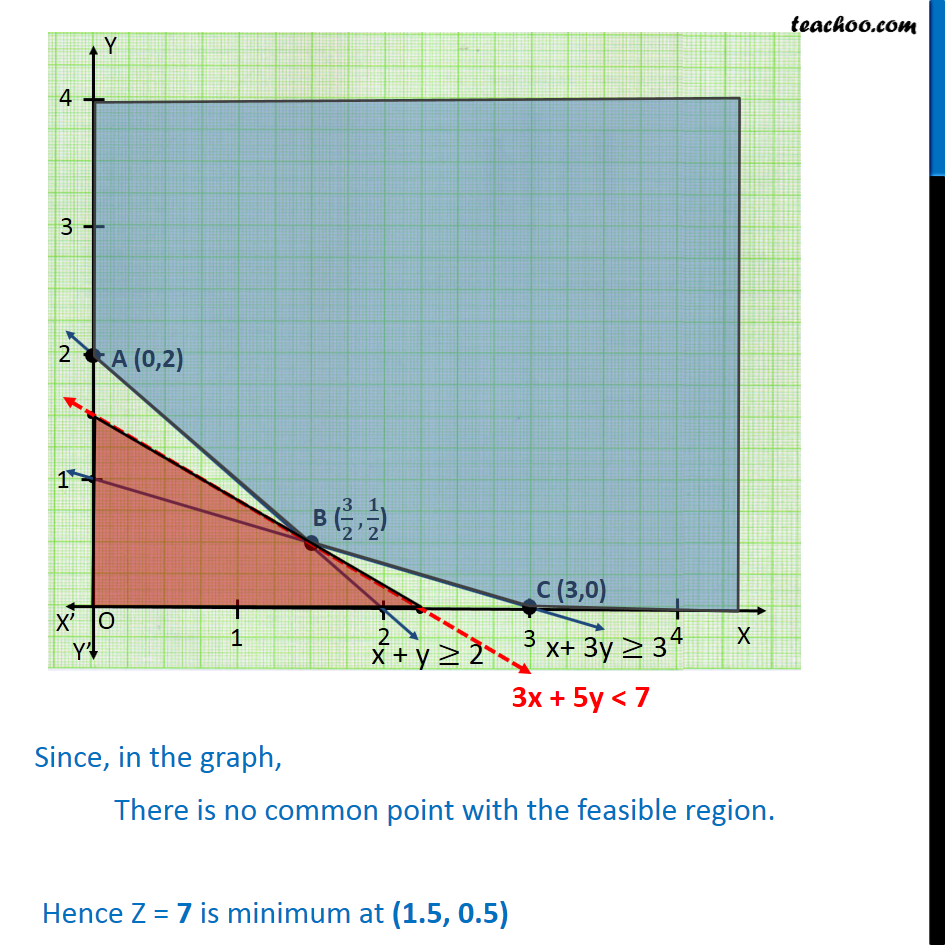Ex 12.1

Chapter 12 Class 12 Linear Programming
Serial order wiseLearn in your speed, with individual attention - Teachoo Maths 1-on-1 Class

### Transcript

Ex 12.1, 4 Solve the following Linear Programming Problems graphically: Minimise Z = 3x + 5y such that x + 3y 3, x + y 2, x, y 0. Minimize Z = 3x + 5y Subject to x + 3y 3 x + y 2 x 0, y 0 As the region that is feasible is unbounded. Hence, 7 may or may not be minimum value of Z. For this we graph inequality,